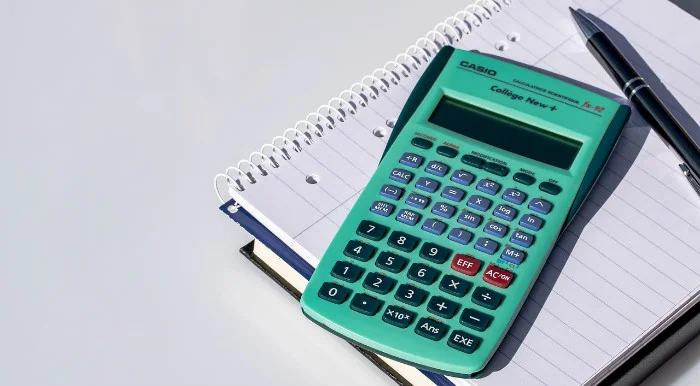# How To Calculate Buy To Let Yield##### If you’ve decided to rent out a property, one thing you’ll need to know is how to calculate buy to let yield.

In fact, even if you haven’t bought a property yet and are still trying to decide whether to buy to let or buy to sell, the yield is essential information.

It’s a simple, yet very important, calculation that will help estimate your expected return from a property over a year.

## What Is Buy To Let Yield?

The buy to let yield is simply the rate of return you can expect to achieve on a property. It helps to visualise how quickly you will make a return on your investment. It will also help you decide whether to rent out or sell it on.

It’s expressed as a percentage of your original purchase price and is based on annual returns. Therefore, a property with a 10% yield would repay your original investment in 10 years time (10% x 10 = 100%). A property with a 5% yield would take twice as long (20 years) to repay your original investment (5% x 20 = 100%).

## The Buy To Let Yield Calculation

When calculating buy to let yield, you only need to know two figures – the expected annual rental income of the property, and the price you paid for it.

If you know these two figures, the formula to calculate it is simply:

(Annual Rental Income ÷ Purchase Price) x 100 = Yield %

In this formula, you do the sum in the brackets first, then multiply the answer by 100 to get the yield. For example, if you purchase a property for £150,000 and you expect to receive £9,000 a year from it in rental income, your calculation would look like this: (9,000 ÷ 150,000) x 100 = 6% Yield.

## Gross Yield Vs Net Yield

The calculation above is a gross yield calculation and is the simplest and most common method for calculating it. It is based on very simplistic figures as it looks only at the price you paid and the total rental income, without taking into account any expenses.

For a more detailed calculation, you would need to work out the net yield of a property. With this, you would deduct any costs from the annual rental income before dividing it by the purchase price. Costs could include things such as letting agent fees, periods when the property is untenanted, maintenance and repair bills, and so on.

Net yield is difficult to calculate accurately in advance since many costs are unknown. It is therefore usually used to look at historic performance rather than to estimate future performance.

## Yield Vs Capital Gain

The yield of a rental property should not be confused with the capital gain. Whereas knowing how to calculate buy to let yield will help you decide whether it is worth renting out a property, knowing the capital gain will help you decide if it is worth selling it.

The capital gain is simply your profit after you have sold it. So it’s your final sales price minus the price you paid for it and all other expenses incurred.

Most investors will look at both the potential capital gain and the expected yield, alongside several other factors, when deciding whether to sell or let an investment.

So, that’s how you calculate yield on a buy to let property. In general, you’ll usually see higher yields on properties in cheaper areas, and lower ones on more expensive ones. However, it does depend on many factors so it’s always worth getting the advice of a good estate agent before you make your final decision.